# Why is 1 not a perfect number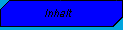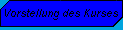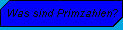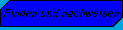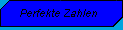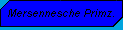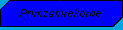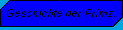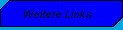### Perfect numbers:

What's a perfect number?

• A number is perfect if the sum of its divisors is * 2. (That is, the divisional sum * 2 results in the number.)
b.) Theorem of Euclid:
• If 2n-1 is a prime number, then 2(n-1)*(2n-1) perfect.
c.) Euler's theorem:
• If a is a perfect even number, then there is an n with:
1. a = 2(n-1)*(2n-1).
2. 2n-1 is a prime number.
Odd perfect numbers:
• It is still unknown whether there are odd perfect numbers!
Proof:
• ts (a) = ts (2(n-1) *2n-1)
= ts (2n-1* ts (2n-1)
=(2n-1)/(2-1)*((2n-1)+1)
=(2n-1)*2n
=(2n-1)*(2*2(n-1))
=2*(2n-1)*(2-1)
= 2 * n

The first six perfect numbers: 6, 28, 496, 8,128, 33,550,336, 8,589,869,056.

1. 6 has the divisors: 1, 2, 3, 6. The divisional sum is 12 because 1 + 2 + 3 + 6 = 12. That is why the number 6 is perfect because 12 = (6 * 2).

6 has the divisors: 1, 2, 3, 6. The divisional sum is 12 because 1 + 2 + 3 + 6 = 12. That is why the number 6 is perfect because 12 = (6 * 2).

28 has the divisors: 1, 2, 4, 7, 14, 28. The divisional sum is 56 because 1 + 2 + 4 + 7 + 14 + 28 = 56. That is why the number 28 is perfect because 56 = (28 * 2).

496 has the partial sum 992. Therefore the number 496 is perfect, because 992 = (496 * 2).

8,128 has the partial sum 1.6256. That is why the number 8,128 is perfect because 16,256 = (8,128 * 2).

33,550,336 has the partial sum 67,100,672. That is why the number 33,550,336 is perfect because 67,100,672 = (33,550,336 * 2).

8,589,869,056 has the partial sum 17,179,738,112. That is why the number 8,589,869,056 is perfect because 17,179,738,112 = (8,589,869,056 * 2).

6 = 2*3
28 = 22*7
496 = 24*31
8.128 = 26*127
33.550.336 = 212*8.191
8.589.869.056 = 216*131.071

© DBG Wiehl, November 16, 1998
& nbsp